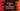# How to sort the array elements in descending order in JavaScript## How to sort the array elements in descending order in JavaScript:

This post will show you how to sort the elements of an array in descending order in JavaScript. JavaScript provides inbuilt methods for arrays to sort the elements. The sort() method sorts the elements in ascending order. There is one more method called reverse() that can be used to reverse the elements of an array. So, we can use sort() and reverse() methods to sort the array elements in descending order.

The sort() method also takes one compare function which can be used to sort the array elements in descending order.

Let’s learn how these methods work with definition.

### sort():

The sort method is used to sort the elements of an array. This method sorts the elements in place and returns the reference to the original array.

The syntax of the sort method is:

``sort()``

As mentioned before, it sorts the array elements in place and returns the reference of the array, which will contain the sorted elements.

We can also pass a function as its parameter to define the sort order. It can be an arrow function, inline function, or reference to a function.

``````sort((first, second) => {})

sort(compareFunction)

sort(function compareFunction(first, second){})``````

The compareFunction is a function to define the sort order. This is optional. If we don’t pass this function, it will convert the array elements to strings and then sorts them as per the character’s Unicode code point value.

• If the compare function returns a number that is greater than 0, it will sort the first element after the second element.
• If it returns a number that is smaller than 0, it will sort the first element before the second element.
• If the number is equal to 0, it will keep the original order of the first and the second element.

### Example 1: JavaScript program to sort the array elements in descending order by using the sort() method:

Let’s use the sort method to sort the array elements in descending order using JavaScript. We will pass the compare function

``````const givenArray = [1, 2, 3, 8, 5, 7];

console.log("Given Array: ", givenArray);
givenArray.sort((first, second) => second - first);

console.log("Array after sort: ", givenArray);``````

Here, givenArray is the original array. We are using the sort function to sort the array in descending order. We are passing an arrow function to the sort method. We can also pass one inline function.

It will print the below output:

``````Given Array:  [ 1, 2, 3, 8, 5, 7 ]
Array after sort:  [ 8, 7, 5, 3, 2, 1 ]``````

Let’s try with alphabets:

``````const givenArray = ["e", "f", "a", "c", "d"];

console.log("Given Array: ", givenArray);
givenArray.sort((first, second) => {
if (first > second) return -1;
if (first < second) return 1;
return 0;
});

console.log("Array after sort: ", givenArray);``````

We have to change the compare function and it returns -1, 1 or 0 by comparing the first and the second values of the array. If you run this program, it will sort the array in descending order.

``````Given Array:  [ 'e', 'f', 'a', 'c', 'd' ]
Array after sort:  [ 'f', 'e', 'd', 'c', 'a' ]``````

### Example 2: JavaScript program to sort the array elements in descending order by using the sort() and reverse() methods:

The reverse() method is an inbuilt method of JavaScript array and it reverses the array in place. It also returns the reference to the original array. We can call the sort() method to sort the elements of an array in ascending order and call reverse() to reverse the elements.

``````const givenArray = ["e", "f", "a", "c", "d"];

console.log("Given Array: ", givenArray);
givenArray.sort().reverse();
console.log("Array after sort: ", givenArray);``````

Since both of these methods modifies the array in place, it will reverse the content of the given array.

If you run the above program, it will print the below output:

``````Given Array:  [ 'e', 'f', 'a', 'c', 'd' ]
Array after sort:  [ 'f', 'e', 'd', 'c', 'a' ]``````

### Example 3: JavaScript program to reverse sort an array of objects:

We can use the sort() method with a custom compare function to sort an array of objects in reverse order. Let’s take a look at the below example:

``````const givenArray = [
{ name: "Alex", id: 10 },
{ name: "Bob", id: 31 },
{ name: "Daisy", id: 2 },
];

console.log("Given Array: ", givenArray);
givenArray.sort((first, second) => {
if (first.id > second.id) return -1;
if (first.id < second.id) return 1;
return 0;
});
console.log("Array after sort: ", givenArray);``````

Here, givenArray is an array of objects. Each object has one name and id properties. The compare function of the sort method compares the id of each object and returns one value similar to the first example. If you run this program, it will sort the objects in descending order of ids.

``````Given Array:  [
{ name: 'Alex', id: 10 },
{ name: 'Bob', id: 31 },
{ name: 'Daisy', id: 2 }
]
Array after sort:  [
{ name: 'Bob', id: 31 },
{ name: 'Alex', id: 10 },
{ name: 'Daisy', id: 2 }
]``````